# Grey Relational Analysis Optimization of Input Parameters for Electrochemical Discharge Drilling of Silicon Carbide by Gunmetal Tool Electrode

Grey Relational Analysis Optimization of Input Parameters for Electrochemical Discharge Drilling of Silicon Carbide by Gunmetal Tool Electrode
Production and Industrial Engineering Department, National Institute of Technology (N.I.T.), Jamshedpur 831014, Jharkhand, India

Corresponding Author Email:
2013rsprod004@nitjsr.ac.in
Page:
239-249
|
DOI:
https://doi.org/10.18280/acsm.440402
March 2020
|
Accepted:
12 May 2020
|
Published:
30 September 2020
| Citation

OPEN ACCESS

Abstract:

The electrochemical discharge machining process (ECDM) is a hybrid advanced technology integrated with electrochemical and electro-discharge processes has used for the manufacturing of non-conducting along with conducting materials. The silicon carbide is non-conducting material which has widely used in various fields such as automobile, aviation, medical, nuclear reactor, and missile. The machining of silicon carbide is a challenging task by using non-conventional along with conventional machining processes due to its physical properties. The current research work shows the machining of Silicon carbide material by using fabricated ECDM machine setup with gunmetal tool material. The Taguchi L27 orthogonal array technique is applied for experimental work. The grey relational analysis optimization is applied for the investigation of optimum input factors for better output responses. The input process factors like electrolyte concentration, applied voltage, and rotation of tool and outcome results such as machined depth and the diameter of hole were checked after drilling of silicon carbide material. The experimental results indicate the electrolyte concentration is the leading factor for diameter of hole and depth of machined hole subsequent to voltage and tool rotation.

Keywords:

ECDM, hole diameter, machined depth, Silicon carbide, gunmetal, grey relational analysis

1. Introduction

Silicon carbide is an amalgamated with carbon and silicon. It is the hardest material having an intense melting point of 2730℃. It is having various properties like high thermal conductivity, resistance to a chemical reaction, high hardness, wear resistance, light-weight, and corrosion resistance. This material is widely used in automobile industries, grinding wheels, refractory linings, cutting tools, aerospace, high-temperature bricks, wear-resistant parts, and heating elements [1, 2]. The cutting of Silicon carbide is a challenging task with the traditional and non-traditional manufacturing processes. The electrochemical discharge machining (ECDM) technology has the amalgam process which can cut Silicon carbide material effectively. This process mingled with the electrochemical process (ECM) along with electro-discharge manufacturing (EDM) . In the year of 1968, Kurafuji et al. had created originally ECDM process and this process was applied for machining of glass . This machining technology can be utilized for performing the process of micro-drilling, machining of cylindrical parts, slicing of glass rods, dressing of micro tools . The application of this process is for producing micro-texturing in microfluidic devices, digital micro-mirror devices, microelectromechanical systems, microscale fabrication of micro-filters . The important parameters of this process are transformation in wettability of the electrode, local Joule heating, hydrodynamic fluctuation, the combination of wettability, hydrodynamic effects, and bubble coalescence. The limitations of this process are machining efficiency, quality, and accuracy. In this process, gravity feed mechanism mostly applied to a workpiece or tool and used for machine workpiece due to the provision of steady connection which causes the machining output responses . In the electrochemical discharge process at a higher level of concentration of electrolyte and applied voltage machining conditions which can accomplish micro-holes precisely and higher material removal rate . The abrasive cathode tools in the ECDM gives maximum machining depth in machining conditions of the extreme applied voltage and concentration of electrolyte . Similarly, The high-quality holes produced with stable material removal can be achieved with the abrasive tool electrode it provides additional cutting action and electrical discharge during the ECDM process . Arab et al. studied the influence of tool surface roughness on the heat-affected zone and overcut during drilling in the glass substrate by ECDM. They found the lower surface roughness tool exhibited a thinner gas film which produces holes with low heat affected zone and overcut . Yang et al. obtained better results as well as reduces machining time by a spherical end tool electrode. They observed that the spherical tool reduces the contact surface between the workpiece and electrode that promotes the flow of electrolyte to the tool end which creates efficient micro-hole drilling . Bhattacharyya et al. found that at higher material removal rate (MRR) was achieved at maximum voltage and higher electrolyte concentration conditions and at a maximum concentration of electrolyte the overcut was higher for aluminium oxide ceramic material . Liu et al. found that when an increase in duty cycle, the concentration of electrolyte, pulse duration, and current can improve the development of discharge action during machining of particulate reinforced metal matrix composite material . Singh et al. produced micro holes on a silicon wafer. The found that hole taper and overcut rises with rise in voltage and reduces with raise in tool feed rate. The chemical action and spark phenomenon at tool is the key principle behind electron chemical erosion of the tool . Laio et al. observed that adding surfactants in the electrolyte can raise current density which produces better quality and a less taper hole on quartz material . Likewise, Dhanvijay et al. studied the influence of flow and without the flow of electrolyte in the ECDM process of fiberglass reinforced plastic material. They showed that moving of electrolyte given regularly fresh supply of electrolyte leads to the increase in hydrogen gas bubbles and therefore increase in material removal rate . Antil et al. choosing a population-based metaheuristic algorithm to achieved maximum material erosion rate of silicon carbide particle/glass fiber–reinforced polymer matrix composite during ECDM machining . The Taguchi L9 orthogonal array method was used to experiments on silicon carbide material using a stainless tool in the ECDM process. In this report, Sarkar et al. found that electrolyte concentration was the most dominant factor for controlling radial overcut and material removal rate . Pawar et al. reported previously ECDM machining of silicon carbide material using brass tool material and optimization of parameters using response surface methodology . In this research work, a developed gravity feed mechanism applied to the ECDM setup has utilized for drilled hole on Silicon carbide with gunmetal tool material. The machining depth and hole diameter were investigated through input process factors such as rotation of tool, voltage, and concentration of electrolyte. The experiments were executed with the help of the Taguchi L27 orthogonal array technique and optimized parameters using grey relational analysis. Grey relational analysis is a good utilization of concerns with fuzzy uncertainty problems and therefore it is mostly used in the engineering field to obtained good outcomes .

2. Basic Mechanism and ECDM Machine Setup

The primary working mechanism of the electrochemical discharge machining is presented in Figure 1. The main components of this machine specifically anode, cathode, and workpiece material are dipped inside the electrolyte solution. In this process, the D.C. voltage is applied among anode and cathode tool electrodes, when voltage goes beyond 30 volts the electrolysis takes place at that instance. However, hydrogen gas bubbles are produced around cathode electrode and oxygen bubbles are produced around the anode tool electrode. At that instant the voltage is raised, suddenly current increases and a huge amount of bubbles are created a bubble layer nearby the anode and cathode. These bubbles made coalesce into a gas film layer nearby the cathode electrode during applied voltage is maximum than that of the critical voltage. At that existent bright spark is observed as well as electrical discharges happened and it causes removal of material .

The manufactured ECDM (Figure 2) machining setup was used to machined silicon carbide. All axes movements were controlled manually. The gravity feed system was applied to travel the silicon carbide plate in an upper movement amid the drilling process. The gunmetal (cathode) tool was mounted to the Z-axis. The cathode was connected to the machine motor shaft and its rotation was monitored using the Arduino Uno board interface to the laptop. The direct current (D.C.) voltage is provided among the anode and cathode electrodes . The taper shape cathode tool electrode (Gunmetal) with diameter to 3 mm diameter of and stainless steel 416 anode tool electrode were used. In experimental research work, NaOH was utilized as electrolyte with machining cycle time for one experiment is 25 minutes.

## f1.png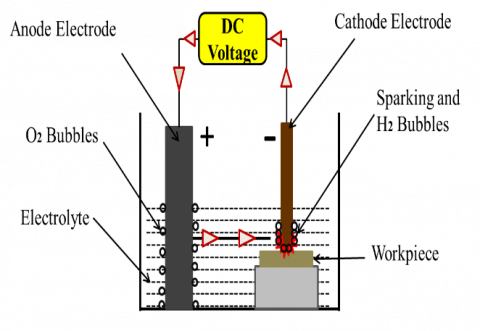Figure 1. Schematic diagram of ECDM process

## f2.jpg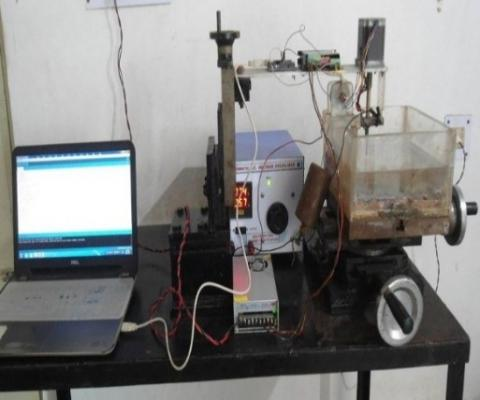Figure 2. ECDM experimental set-up

3. Experimental Conditions

The electrochemical discharge machining process was used to produce micro-holes on silicon carbide material having a size of 150×150×10 mm3. The experimental method has been done by using Taguchi L27 orthogonal technique. The reason for selecting Taguchi L27 orthogonal is because of deciding three parameters and their three various levels with two ways of interconnection hence, eighteen all degrees of freedom. So, the L27 orthogonal array method was applied for experimental work which provides 26 degrees of freedom. In current research work, applied voltage, tool rotation, and concentration of electrolytes were considered as input process factors and results are machined depth and drilled hole diameter. The input process factors with their specific levels depicted in Table 1 .

Table 1. Process parameters and their levels

 Levels Parameters Voltage (V) Rotation of Tool (RPM) Electrolyte Concentration (%) 1 70 0 10 2 80 10 15 3 90 50 20

In this research work signal to noise (S/N) ratio criteria was utilized as an analysis of experimental data. The signal represents the influence of every parameter on the outcome results, however, noise represents the measure of the influence of irregularity as of average outcome results. In this research, the diameter of hole was treated as nominal is best because the average diameter of hole outcome responses were examined and the diameter of hole 3.25 mm was assigned as the targeted nominal value. Similarly, in case of depth of machined hole higher the better precedent was selected. The S/N ratio was assessed with the help of given formula which is presented below in Eq. 1 and Eq. 2 .

For larger is better:

$S/N=-10\mathop{\log }_{10}\left( \frac{1}{n}\sum\limits_{i=1}^{n}{\frac{1}{\mathop{y}_{i}^{2}}} \right)$           (1)

$S/N=-10\mathop{\log }_{10}\left( \frac{1}{n}\sum\limits_{i=1}^{n}{\mathop{\sigma }^{2}} \right)$      (2)

where, n represents the number of measurements and yi is the measured valueFor nominal is best:

It is observed that when the voltage applied between cathode and anode the bubbles generated because of electrochemically liberated H2 and water vapour created through ohmic heating at the cathode electrode interface. The isolation among the electrode and the electrolyte occurred. The isolation among the electrolyte and the electrode leads to discharge because of switching e.m.f. The large electric field source sparks inside the gas bubble isolating the tip. The spark has arisen between the inside surface of the electrolyte and the tip of the tool electrode. At the instant during sparks ensues, an avalanche of electrons initiated by ionization flow towards workpiece kept around 20 μm distance away from the tooltip. It is a drifting phenomenon of the electron avalanche and therefore this process is discrete and repetitive. The bombardment of electrons on the silicon carbide material surface results in intense heating due to the discharge process and therefore material removal takes place [25, 26]. Table 2 indicates the Taguchi L27 experimental conditions and their individual results. The individual sparking conditions for each experiment are shown in Table 3 during the machining of silicon carbide material. The intensity of spark was raised when the raising of concentration of electrolyte and voltage which produces enlarge in diameter of hole and machined depth.

The individual microscopic images of drilled holes on silicon carbide material with gunmetal tool electrode by the ECDM process are shown in Table 4. The tooltip can generate an indent at the starting of machining, which indications the tool in ECDM. The distribution of discharge energy is more uniform when the electrode rotates. On the other hand, a high tool rotation rate can deteriorate the discharge effect, thus dropping excessive sparks. A higher rotational rate avoids the discharge focuses on the same points and improves the dimensional precision of micro-holes. The rotation of the electrode clearly enhances the roundness of a micro-hole. When electrolysis is confined at the tooltip, the maximum number of the bubbles accomplished at the active tool exterior magnificently consolidate into a spherical dielectric gas film. The gas film thickness based on the geometry of the tool, i.e. exposure length and the tool radius, which is adequately equal to the tool depth in the electrolyte. It is observed that machining depth rises with the rise in applied voltage this is due to field emission law [8, 27, 28].

Table 2. Observations of experimental results of Silicon carbide through gunmetal tool electrode

 No. Conc. of Elec. (%) Voltage (V) Rotation (rpm) Diameter of Hole (mm) Depth of Machined Hole (mm) 1 10 70 0 1.96 0.10 2 10 70 20 2.20 0.10 3 10 70 50 2.78 0.15 4 10 80 0 2.54 0.15 5 10 80 20 2.69 0.20 6 10 80 50 2.87 0.20 7 10 90 0 2.70 0.22 8 10 90 20 2.82 0.26 9 10 90 50 3.21 0.30 10 15 70 0 2.88 0.20 11 15 70 20 3.11 0.32 12 15 70 50 3.04 0.30 13 15 80 0 3.28 0.25 14 15 80 20 3.42 0.45 15 15 80 50 3.50 0.50 16 15 90 0 3.47 0.54 17 15 90 20 3.72 0.59 18 15 90 50 3.85 0.67 19 20 70 0 3.10 0.42 20 20 70 20 3.35 0.50 21 20 70 50 3.76 0.68 22 20 80 0 3.83 0.63 23 20 80 20 4.02 0.71 24 20 80 50 4.15 0.75 25 20 90 0 3.91 0.71 26 20 90 20 4.32 0.79 27 20 90 50 4.85 0.88

Table 3. Spark intensities for each experimental conditions

Gunmetal Tool Electrode Experiments

Rotation (rpm)

Volt.

Conc.

0

20

50

(V)

(%)

## 31.png## 32.png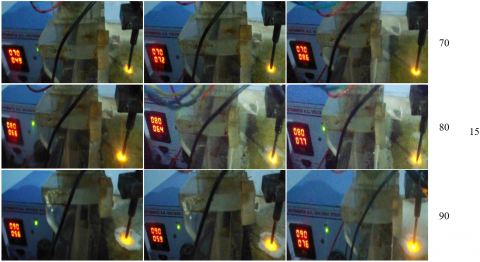## 33.png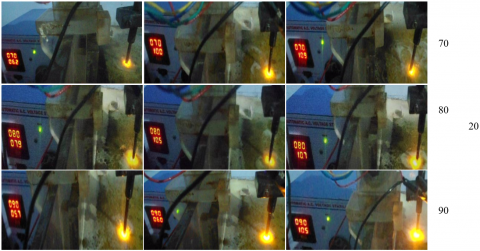Table 4. Microscopic images of drilled hole on silicon carbide material through gunmetal tool

Gunmetal Tool Experimental Results

Rotation (r/min)

Volt.

(V)

Conc.

(%)

0

20

50

## 41.png## 42.png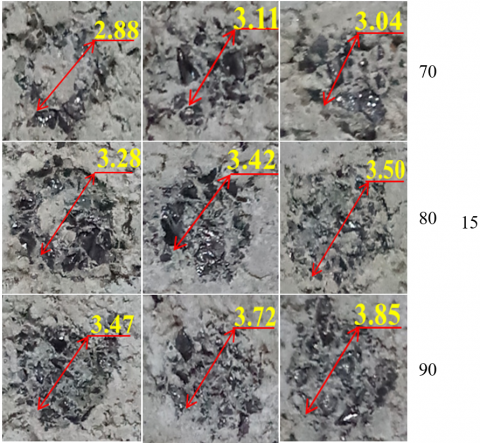## 43.png4. Analysis of Machined Depth of Silicon Carbide Using Gunmetal Tool Material

Here, the influence of machining parameters for the machined hole of Silicon carbide through the gunmetal tool is explained. The ANOVA statistical method was utilized to evaluate how much influence of the input factors. The output response table for the signal to noise (S/N) ratios, higher the better criteria for machined depth was used for ranking of the input factors. The influence graph for S/N ratios for output response of machined depth is also explained.

4.1 ANOVA for depth of machined hole

The ANOVA for the depth of the machined hole for Silicon carbide through the gunmetal tool electrode with the condition of higher the better is shown in Table 5. It shows that the largest F-value 165.93 was found for the concentration of electrolyte which indicates the most powerful parameters as compared to the remaining two input factors. The depth of machined hole raised with raising the concentration of electrolytic from 15 to 20 percentage. It is also noticed that the depth of machined hole outcome responses were identical trends to earlier published papers by researchers [28-30]. The three input factors were a noticeable effect on the depth of machined hole due to the reason of P values are lower than 0.05. The concentration of electrolyte is provided 72.17%, the applied voltage is provided 17.96%, and tool rotation is provided 5.53% successively. The response Table 6 for the S/N ratios of the depth of machined hole of silicon carbide material through the gunmetal tool which represents that concentration of the electrolyte is the first rank be subsequent to applied voltage and tool rotation.

Table 5. Analysis of variance (ANOVA) table for depth of machined hole through gunmetal tool electrode

 Source DF Adj. SS Adj. MS F Value P Value Percentage Contribution Applied Voltage 2 0.267 0.133 41.29 0.00 17.96 Conc. of Electrolyte 2 1.071 0.535 165.93 0.00 72.17 Rotation Speed 2 0.082 0.041 12.71 0.00 5.53 Error 20 0.064 0.003 4.34 Total 26 1.484 100

Table 6. Response for signal to noise (S/N) ratios of depth of machined hole

 Level Voltage Electrolyte Concentration Rotation Speed 1 -11.969 -15.136 -10.614 2 -8.769 -8.083 -8.682 3 -6.095 -3.614 -7.537 Delta 5.874 11.522 3.077 Rank 2 1 3

4.2 Effect on the depth of machined hole through gunmetal tool

Figure 3 i.e. main effect plot indicates that the depth of machined hole raises noticeably with the rise in the concentration of electrolyte, voltage, and tool rotation. The highest depth of the machined hole was found at input parameters conditions of concentration of electrolyte of 20% applied voltage 90 V, and rotation of the tool of 50 r/min. The identical graphical results were obtained to earlier research papers for glass and Soda-lime glass material [31, 32]. The material erosion of Silicon carbide raises with the rise in voltage and electrolyte concentration .

## ff1.png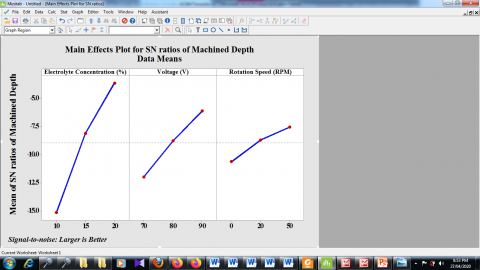Figure 3. Main effect plot for the machined depth of Silicon carbide through Gunmetal tool material

## ff2.png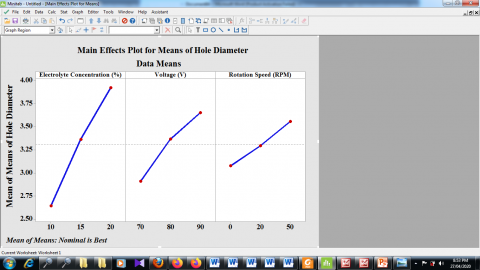Figure 4. Diameter of hole plot for Silicon carbide through gunmetal tool electrode

5. Analysis for the Diameter of Hole of Silicon Carbide Through Gunmetal Tool

This section discussed the analysis of variance table for the diameter of hole for silicon carbide material through gunmetal tool. The nominal is better criteria is chosen for the response table for means of diameter hole. Also, elucidated the main effect graph for the diameter of hole of silicon carbide through the gunmetal electrode.

5.1 ANOVA for the diameter of hole

The nominal is the best criteria applied to the diameter of hole of silicon carbide through gunmetal tool material and analysis of variance results for given conditions is shown in Table 7. It shows that the largest F value 160.80 found for the concentration of electrolyte which represents it is the powerful parameter compared to the remaining two input parameters. The p values denote that all input factor values are less than 0.05 which shows that all parameters are noticeable. The concentration of the electrolyte is provided 64.80%, the applied voltage is 22.00% and tool rotation is provided 9.17% contributions respectively. The results achieved has very much similar to already published papers by authors for the diameter of hole and radial overcut for glass workpiece material [8, 9, 28, 30, 34]. The response table for means of the diameter of hole on silicon carbide through the gunmetal tool is shown in Table 8. It represents that the concentration of electrolyte is the first rank be subsequent to applied voltage and tool rotation.

Table 7. ANOVA table for hole diameter of Silicon carbide through Gunmetal electrode

 Source DF Adj. SS Adj. MS F Value P Value Percentage Contribution Voltage 2 2.517 1.259 54.60 0.000 22.00 Electrolyte Conc. 2 7.413 3.707 160.80 0.000 64.80 Rotation Speed 2 1.049 0.525 22.76 0.000 9.17 Error 20 0.461 0.0231 4.03 Total 26 11.440 100

Table 8. Response table for the mean of hole diameter of Silicon carbide through gunmetal tool

 Level Voltage Electrolyte Concentration Rotation Speed 1 2.909 2.641 3.074 2 3.367 3.363 3.294 3 3.650 3.921 3.557 Delta 0.741 1.280 0.482 Rank 2 1 3

5.2 Effect of machining factors on the diameter of hole

The diameter of hole rises noticeably with the rise in the concentration of electrolyte, voltage and tool rotation linearly which is represented in Figure 4. The large hole diameter accomplished at machining factors combination of applied voltage 90 V, the concentration of electrolyte 20% and the tool rotation of 50 r/min. The identical graphical results were accomplished in the earlier research paper for glass and Soda-lime glass materials [9, 30].

6. Grey Relational Analysis of Silicon Carbide Output Responses Through Gunmetal Tool

The grey relational analysis theory was firstly presented by Prof. Deng in the year 1982 has been extensively utilized to distinct applications. It is appropriate for cope with uncertain information, incomplete and poor data as well as it is a multi-objective optimization technique. This theory is applicable to determine problems with convoluted inter-relationships among variables and multiple parameters [35, 36]. The “grey” word in the term can be described as an attribute among black and white. Thus, “black” indicates desired data is not specifically available; on the contrary “white” means desired data is specifically available. “Grey” theory implied that relation among black with white. Thus, the grey theory supports only the intrinsic structure having limited data [37, 38]. The desirability criterion optimum ECDM drilling process through the gunmetal tool is the maximum depth of machined hole and the nominal diameter of hole. The responses quality characteristics i.e. machining depth and hole diameter were analyzed for experimental results preprocessing depends upon the ‘higher the better’ and “nominal the best’ criterion.

6.1 The calculating process of grey relational analysis

The following are steps used for the calculation of grey relational analysis.

Step I: Find out the assessed statistics and transform the new statistics. Then, to exclude the different measurements of the new statistics and transform them into statistics that can be related to all statistics.

Step II: Calculate the statistics sequence for reference. Then the reference sequence was chosen depending on the particular application.

Step III: Evaluate the complete variance and the maximum and minimum values of the related factor among the reference statistics sequence and the statistics sequence of every assessed object then evaluate the interrelationship coefficients of both sequences

Step IV: Evaluate the grey correlation degree. The calculation of mean of the correspondence coefficient among the statistics ratio of every position and the related factors of the reference order is assessed as the grey correlation degree of both orders to reveal the connection among the reference orders as well as the evaluation objects.

Step V: Locate entirely grey correspondence degrees in the related to order from small to large and from large to small .

6.2 Data pre-processing

In the grey relational analysis optimization method, response results pre-processing is needed as the assortment and entity in one experimental result sequence can vary against the remaining results. It is required while the sequence scatters extent is huge. In this method, the data is transferring the original order to a proportionate order. Thus, the output responses are normalized in the span from zero to one. The various approaches were used for investigating experimental results pre-processing as the grey relational analysis. These approaches are given below .

1. The experimental data is transformed into normalized.
2. The grey relational coefficient was assessed with the normalized experimental results to definite the correlation among the actual and desired experimental results information.
3. The grey relational grade has estimated through equating the grey relational coefficient correspondent to all output results.
4. The overall estimation of the multiple output responses is established on the grey relational grade. Correspondingly, optimization of the convoluted multiple output responses may be transformed within the optimization of an individual grey relational grade.

6.3 Grey relational generating

The original order along with the order of assessment usually defined as $x_{0}$ (k) and $x_{i}$ (k), i=1, 2, 3...., m; k=1, 2, 3..., n accordingly. While, m is the total number of experiments, where n is the number of all experimental data. The $x_{i}^{*}$  (k) is the value after Grey relation generating. If the objective value of the original order is larger the better then the original order is normalized with respect to Eq. 3 is given below [35, 37, 40]. In the data preprocessing step, Eq. 3 is used to obtain normalized results for machining depth. Eq. 5 is used to evaluate normalized results for hole diameter.

$x_{i}^{*}(k)=\frac{x_{i}^{0}(k)-\min x_{i}^{0}(k)}{\max x_{i}^{0}(k)-\min x_{i}^{0}(k)}$           (3)

$x_{i}^{*}(k)=\frac{\max x_{i}^{0}(k)-x_{i}^{0}(k)}{\max x_{i}^{0}(k)-\min x_{i}^{0}(k)}$           (4)

In the case, there is a target value the original sequence is normalized as specified below Eq. 5. OB is the target value.Similarly, if it is 'smaller-the-better', then the original sequence is normalized as Eq. 4.

$x_{i}^{*}(k)=1-\frac{\left| x_{i}^{0}(k)-OB \right|}{\max \{x_{i}^{0}(k)-OB;OB-\min x_{i}^{0}(k)\}}$     (5）

Here, $\Delta_{0 i}(k)$  is a deviation order between $x_{0}^{*}(k)$ reference sequence and $x_{i}^{*}(k)$ comparable sequence. This deviation sequence is assessed with the help of Eq. 6.

6.4 Deviation sequence

${{\Delta }_{0i}}(k)=\left| x_{0}^{*}(k)-x_{i}^{*}(k) \right|$         （6）

Likewise, the largest deviation and the minimal deviation are evaluated as in Eq. 7 and Eq. 8.

$\mathop{\Delta }_{\max }=\underset{\forall j\in i}{\mathop{\max }}\,\underset{\forall k}{\mathop{\max }}\,\left| x_{0}^{*}(k)-x_{j}^{*}(k) \right|$               （7）

$\mathop{\Delta }_{\min }=\underset{\forall j\in i}{\mathop{\min }}\,\underset{\forall k}{\mathop{\min }}\,\left| x_{0}^{*}(k)-x_{j}^{*}(k) \right|$        （8）

$\sum_{k=1}^{n} \beta_{k}=1$  here, the Grey relational grade $\gamma\left(x_{0}^{*}, x_{i}^{*}\right)$ denotes the level of correlation among the reference and the comparability order. The maximum grey relational grade denotes enhance product quality; thus, based on grey relational grade, the factor influence can be assessed and the optimal level for an individual factors can also be evaluated [41, 42].

6.5 Grey relational coefficient

Grey relational coefficient is utilized for evaluating how close $x_{0}^{*}(k)$  is to $x_{i}^{*}(k)$ . The bigger the grey relational coefficient, the closer $x_{0}^{*}(k)$  and $x_{i}^{*}(k)$ . The grey relational coefficient can be evaluated by Eq. 9. The identification coefficient ζ taking a value 0.5 was substituted in Eq. 9 to find out the grey relational coefficient for the individual experiment.

$\gamma \left[ x_{0}^{*}(k),x_{i}^{*}(k) \right]=\frac{\Delta \min +\xi \Delta \max }{\Delta {{x}_{i}}(k)+\xi \Delta \max }$     （9）

where, $\xi$  is the distinguishing coefficient in [0, 1].

$0\text{}\gamma \left[ x_{0}^{*}(k),x_{i}^{*}(k) \right]\le 1$                （10）

Table 9. Grey relational analysis of Silicon carbide using Gunmetal tool material

 No. Normalised Value Deviation sequence Grey relation coefficient Grade Rank Machined Depth Hole Diameter Machined Depth Hole Diameter Machined Depth Hole Diameter 1 0.0000 0.0000 1.0000 1.0000 0.3333 0.3333 0.3333 27 2 0.0000 0.0830 1.0000 0.9170 0.3333 0.3529 0.3431 26 3 0.0641 0.2837 0.9359 0.7163 0.3482 0.4111 0.3797 24 4 0.0641 0.2007 0.9359 0.7993 0.3482 0.3848 0.3665 25 5 0.1282 0.2526 0.8718 0.7474 0.3645 0.4008 0.3827 23 6 0.1282 0.3149 0.8718 0.6851 0.3645 0.4219 0.3932 21 7 0.1538 0.2561 0.8462 0.7439 0.3714 0.4019 0.3867 22 8 0.2051 0.2976 0.7949 0.7024 0.3861 0.4158 0.4010 19 9 0.2564 0.4325 0.7436 0.5675 0.4021 0.4684 0.4352 15 10 0.1282 0.3183 0.8718 0.6817 0.3645 0.4231 0.3938 20 11 0.2821 0.3979 0.7179 0.6021 0.4105 0.4537 0.4321 16 12 0.2564 0.3737 0.7436 0.6263 0.4021 0.4439 0.4230 18 13 0.1923 0.4567 0.8077 0.5433 0.3824 0.4793 0.4308 17 14 0.4487 0.5052 0.5513 0.4948 0.4756 0.5026 0.4891 13 15 0.5128 0.5329 0.4872 0.4671 0.5065 0.5170 0.5117 11 16 0.5641 0.5225 0.4359 0.4775 0.5342 0.5115 0.5229 10 17 0.6282 0.6090 0.3718 0.3910 0.5735 0.5612 0.5673 9 18 0.7308 0.6540 0.2692 0.3460 0.6500 0.5910 0.6205 6 19 0.4103 0.3945 0.5897 0.6055 0.4588 0.4523 0.4555 14 20 0.5128 0.4810 0.4872 0.5190 0.5065 0.4907 0.4986 12 21 0.7436 0.6228 0.2564 0.3772 0.6610 0.5700 0.6155 7 22 0.6795 0.6471 0.3205 0.3529 0.6094 0.5862 0.5978 8 23 0.7821 0.7128 0.2179 0.2872 0.6964 0.6352 0.6658 4 24 0.8333 0.7578 0.1667 0.2422 0.7500 0.6737 0.7118 3 25 0.7821 0.6747 0.2179 0.3253 0.6964 0.6059 0.6511 5 26 0.8846 0.8166 0.1154 0.1834 0.8125 0.7316 0.7721 2 27 1.0000 1.0000 0.0000 0.0000 1.0000 1.0000 1.0000 1

Table 10. Response table for average grey relational grade

 Parameter Level 1 Level 2 Level 3 Max-min Rank Applied voltage 0.4305 0.5055 0.5952 0.1647 2 Electrolyte Conc. 0.3802 0.4879 0.6631 0.2829 1 Tool Rotation 0.4598 0.5058 0.5656 0.1058 3

Grey relational grade is the addition of Grey Relational coefficients and it is denoted in Eq. 11.

$\gamma (x_{0}^{*},x_{i}^{*})=\sum\limits_{k=1}^{n}{\mathop{\beta }_{k}\gamma \left[ x_{0}^{*}(k),x_{i}^{*}(k) \right]}$     （11）

The individual ranking of experiments was evaluated by using grey relational grade equation number 11. The normalized values, deviation sequence, grey relational coefficient, grey relational grade, and ranking has been depicted in Table 9. The Taguchi grey relational analysis approach was used to attained grey relational grades to achieve the combination of the optimal factors for multi-objective optimization. The values of the grey relational grade for voltage, electrolyte concentration and tool rotation at level 1 to level 3 were calculated which is shown in Table 10 [41-43].

The mean of the chosen grey relational grade was evaluated using the following formula no. 12 for electrolyte concentration level 1 and assembled to make a response table which is shown in Table 10. The grades in the response table deal with to check the relationship among the comparability order and reference order of grey relational analysis. The maximum values of the average grey relational grade imply a strong correlation. Hence, from Table 10, it is the combination of optimal parameters which maximize all response. Table 10 shows that maximum grey relational grades exist for level 3 of applied voltage, electrolyte concentration and tool rotation means the optimal combination is the voltage of 90 V, electrolyte concentration of 20 % and tool rotation of 50 rpm.

Electrolyte..Conc..(L.1)\text{=}\frac{\begin{align}& \text{0}\text{.3333}+0.3431+0.3797+0.3665+0.3827+ \\& 0.3932+0.3867+0.401+0.4352 \\ \end{align}}{9}        (12)

7. Conclusions

The fabricated ECDM machine setup was used to machined silicon carbide material. In this research work, the output responses such as depth of machined hole and diameter of the hole were investigated by in view of the three input parameters like electrolyte concentration, applied voltage, and rotation of the tool. The experiments were executed with the ECDM on the silicon carbide workpiece through the gunmetal electrode. The grey relational multiobjective optimization technique coupled with Taguchi L27 was utilized to identify the optimum input factors for better output responses. The grey relational multiobjective optimization technique results show that the concentration of electrolyte was the most powerful factor for the depth of machined hole and diameter of hole subsequent to voltage and rotation of the tool. The optimum input machining parameters for the maximum depth of machined hole and the nominal value for the diameter of hole are voltage 90 V, the concentration of electrolyte 20%, and tool rotation 50 rpm. Further, present research findings on the ECDM technology can be utilized for different machining operations on Silicon carbide material according to requirements of various engineering fields with the help of various types of tool materials. The different types of optimization techniques can also be used to optimized output responses.

Nomenclature
 ECDM Electrochemical discharge machining ECM Electrochemical machining EDM S/N ratios Electro discharge machining Signal to Noise ratios ANOVA Analysis of variance
References

 Eom, J.H., Kim, Y.W., Raju, S. (2013). Processing and properties of macroporous silicon carbide ceramics: A review. Journal of Asian Ceramic Societies, 1(3): 220-242. https://doi.org/10.1016/j.jascer.2013.07.003

 Pawar, P., Ballav, R., Kumar, A. (2017). Machining Processes of silicon carbide: A review. Reviews on Advanced Materials Science, 51(1): 62-76.

 Goud, M., Sharma, A.K., Jawalkar, C. (2016). A review on material removal mechanism in electrochemical discharge machining (ECDM) and possibilities to enhance the material removal rate. Precision Engineering, 45: 1-17. https://doi.org/10.1016/j.precisioneng.2016.01.007

 Kurafuji, H., Suda, K. (1968). Electrical discharge drilling of glass. Annals of the CIRP, 16: 415–419.

 Singh, T., Dvivedi, A. (2016). Developments in electrochemical discharge machining: A review on electrochemical discharge machining, process variants and their hybrid methods. International Journal of Machine Tools & Manufacture, 105: 1-13. https://doi.org/10.1016/j.ijmachtools.2016.03.004

 Pawar, P., Ballav, R., Kumar, A. (2018). FEM Analysis of Different Materials Based on Explicit Dynamics ANSYS in Electrochemical Discharge Machine. In: U. Dixit, R. Kant. (eds), Simulations for Design and Manufacturing. Lecture Notes on Multidisciplinary Industrial Engineering, Springer, Singapore, pp. 231-258. https://doi.org/10.1007/978-981-10-8518-5_9

 Wuthrich, R., Fascio, V. (2005). Machining of non-conducting materials using electrochemical discharge phenomenon-an overview. International Journal of Machine Tools & Manufacture, 45(9): 1095-1108. https://doi.org/10.1016/j.ijmachtools.2004.11.011

 Yang, C.T., Ho, S.S., Yan, B.H. (2001). Micro hole machining of borosilicate glass through electrochemical discharge machining (ECDM). Key Engineering Materials, 196: 149-166. https://doi.org/10.4028/www.scientific.net/KEM.196.149

 Jain, V.K., Choudhury, S.K., Ramesh, K.M. (2002). On the machining of alumina and glass. International Journal of Machine Tools and Manufacture, 42(11): 1269-1276. https://doi.org/10.1016/S0032-3861(02)00241-0

 Chak, S.K., Rao, P.V. (2007). Trepanning of Al2O3 by electro-chemical discharge machining (ECDM) process using abrasive electrode with pulsed DC supply. International Journal of Machine Tools and Manufacture, 47(14): 2061-2070. https://doi.org/10.1016/j.ijmachtools.2007.05.009

 Arab, J., Kannojia, H.K., Dixit, P. (2019). Effect of tool electrode roughness on the geometric characteristics of through-holes formed by ECDM. Precision Engineering, 60: 437-447. https://doi.org/10.1016/j.precisioneng.2019.09.008

 Yang, C.K., Wu, K.L., Hung, J.C., Lee, S.M., Lin, J.C., Yan, B.H. (2011). Enhancement of ECDM efficiency and accuracy by spherical tool electrode. International Journal of Machine Tools and Manufacture, 51(6): 528-535. https://doi.org/10.1016/j.ijmachtools.2011.03.001

 Bhattacharyya, B., Doloi, B.N., Sorkhel, S.K. (1999). Experimental investigations into electrochemical discharge machining (ECDM) of non-conductive ceramic materials. Journal of Materials Processing Technology, 95(1-3): 145-154. https://doi.org/10.1016/S0924-0136(99)00318-0

 Liu, J.W., Yue, T.M., Guo, Z.N. (2010). An analysis of the discharge mechanism in electrochemical discharge machining of particulate reinforced metal matrix composites. International Journal of Machine Tools and Manufacture, 50(1): 86-96. https://doi.org/10.1016/j.ijmachtools.2009.09.004

 Singh, M., Singh, S., Kumar, S. (2019). Experimental investigation for generation of micro-holes on silicon wafer using electrochemical discharge machining process. Silicon, 1-7. https://doi.org/10.1007/s12633-019-00273-8

 Laio, Y.S., Wu, L.C., Peng, W.Y. (2013). A study to improve drilling quality of electrochemical discharge machining (ECDM) process. Procedia CIRP, 6: 609-614. https://doi.org/10.1016/j.procir.2013.03.105

 Dhanvijay, M.R., Kulkarni, V.A., Doke, A. (2019). Experimental investigation and analysis of electrochemical discharge machining (ECDM) on fiberglass reinforced plastic (FRP). Journal of The Institution of Engineers (India): Series C, 100(5): 763-769. https://doi.org/10.1007/s40032-019-00524-y

 Antil, P., Singh, S., Singh, S., Prakash, C., Pruncu, C.I. (2019). Metaheuristic approach in machinability evaluation of silicon carbide particle/glass fiber–reinforced polymer matrix composites during electrochemical discharge machining process. Measurement and Control, 52(7-8): 1167-1176. https://doi.org/10.1177/0020294019858216

 Sarkar, B.R., Doloi, B., Bhattacharyya, B. (2017). Investigation on electrochemical discharge micro-machining of silicon carbide. International Journal of Materials Forming and Machining Processes (IJMFMP), 4(2): 29-44. https://doi.org/10.4018/IJMFMP.2017070103

 Pawar, P., Ballav, R., Kumar, A. (2019). Analysis of machining for silicon carbide on electrochemical discharge machining with brass tool. International Journal of Modern Manufacturing Technologies, 11(1): 86-94.

 Shang, H. (2020). Model and algorithms of enterprise informatization software selection based on grey relational analysis. Ingénierie des Systèmes d’Information, 25(1): 107-112. https://doi.org/10.18280/isi.250114

 Pawar, P., Ballav, R., Kumar, A. (2018). Development and manufacturing of arduino based electrochemical discharge machine. Journal of Machine Engineering, 18(1): 45-60. https://doi.org/10.5604/01.3001.0010.8822

 Pinar, A.M., Uluer, O., Kırmaci, V. (2009). Optimization of counter flow Ranque–Hilsch vortex tube performance using Taguchi method. International Journal of Refrigeration, 32(6): 1487-1494. https://doi.org/10.1016/j.ijrefrig.2009.02.018

 Roy, A., Nath, N., Nedelcu, D. (2017). Experimental investigation on variation of output responses of as cast TiNiCu shape memory alloys using wire EDM. International Journal of Modern Manufacturing Technologies, 9(1): 90–101.

 Basak, I., and Ghosh, A. (1997). Mechanism of material removal in electrochemical discharge machining: a theoretical model and experimental verification. Journal of Materials Processing Technology, 71(3): 350-359. https://doi.org/10.1016/S0924-0136(97)00097-6

 Kulkarni A.V. (2007). Electrochemical discharge machining process. Defence Science Journal, 57(5): 765-770. https://doi.org/10.14429/dsj.57.1812

 Han, M.S., Min, B.K., Lee, S.J. (2009). Geometric improvement of electrochemical discharge micro-drilling using an ultrasonic-vibrated electrolyte. Journal of Micromechanics and Microengineering, 19(6): 065004. https://doi.org/10.1088/0960-1317/19/6/065004

 Wei, C., Ni, J., Hu, D. (2010). Electrochemical discharge machining using micro-drilling tools. Transactions of NAMRI/SME, 38: 105-111.

 Wuthrich, R., Spaelter, U., Wu, Y., Bleuler, H. (2006). A systematic characterization method for gravity-feed micro-hole drilling in glass with spark assisted chemical engraving (SACE). Journal of Micromechanics and Microengineering, 16(9): 1891-1896. https://doi.org/10.1088/0960-1317/16/9/019

 Razfar, M.R., Ni, J., Behroozfar, A., Lan, S. (2013). An investigation on electrochemical discharge micro-drilling of glass. In ASME 2013 International Manufacturing Science and Engineering Conference collocated with the 41st North American Manufacturing Research Conference, V002T03A013-V002T03A013. https://doi.org/10.1115/MSEC2013-1135

 Paul, L., Hiremath, S. (2014). Evaluation of process parameters of ECDM using grey relational analysis. Procedia Materials Science, 5: 2273-2282. https://doi.org/10.1016/j.mspro.2014.07.446

 Goud, M., Sharma, A.K. (2017). On performance studies during micromachining of quartz glass using electrochemical discharge machining. Journal of Mechanical Science and Technology, 31(3): 1365-1372. https://doi.org/10.1007/s12206-017-0236-8

 Chak, S.K., Rao, P.V. (2014). Machining of SiC by ECDM process using different electrode configurations under the effect of pulsed DC. International Journal of Manufacturing Technology and Management, 28 (1/2/3): 39-59. https://doi.org/10.1504/IJMTM.2014.064629

 Gao, C., Liu, Z., Li, A. (2014). Study of micro drilling on pyrex glass using spark assisted chemical engraving. Micro and Nanosystems, 6(1): 26-33. https://doi.org/10.2174/1876402905666131112201358

 Kuo, Y., Yang, T., Huang, G.W. (2008). The use of grey relational analysis in solving multiple attribute decision-making problems. Computers and Industrial Engineering, 55(1): 80-93. https://doi.org/10.1016/j.cie.2007.12.002

 Porwal, R.K., Yadava, V., Ramkumar, J. (2013). Multi-objective optimization of hole drilling electrical discharge micromachining process using grey relational analysis coupled with principal component analysis. Journal of The Institution of Engineers (India): Series C, 94(4): 317-325. https://doi.org/10.1007/s40032-013-0078-9

 Ertugrul, I., Oztas, T., Ozcil, A., Oztas, G.Z. (2016). Grey relational analysis approach in academic performance comparison of university: A case study of Turkish universities. European Scientific Journal, Special Edition: 128-139.

 Dhal, P.R., Datta, S., Mahapatra, S.S. (2011). Flexible manufacturing system selection based on grey relation under uncertainty. International Journal of Services and Operations Management, 8(4): 516-534. https://doi.org/10.1504/IJSOM.2011.039667

 Wang, X.L. (2019). Application of grey relation analysis theory to choose high reliability of the network node. In Journal of Physics: Conference Series, IOP Publishing, 1237(3): 032056. https://doi.org/10.1088/1742-6596/1237/3/032056

 Hasani, H., Tabatabaei, S.A., Amiri, G. (2012). Grey relational analysis to determine the optimum process parameters for open-end spinning yarns. Journal of Engineered Fibers and Fabrics, 7(2): 81-86. https://doi.org/10.1177/155892501200700212

 Haq, A.N., Marimuthu, P., Jeyapaul, R. (2008). Multi response optimization of machining parameters of drilling Al/SiC metal matrix composite using grey relational analysis in the Taguchi method. The International Journal of Advanced Manufacturing Technology, 37(3-4): 250-255. https://doi.org/10.1007/s00170-007-0981-4

 Jung, J.H., Kwon, W.T. (2010). Optimization of EDM process for multiple performance characteristics using Taguchi method and Grey relational analysis. Journal of Mechanical Science and Technology, 24(5): 1083-1090. https://doi.org/10.1007/s12206-010-0305-8

 Antil, P., Singh, S., Manna, A. (2018). Electrochemical discharge drilling of SiC reinforced polymer matrix composite using Taguchi’s grey relational analysis. Arabian Journal for Science and Engineering, 43(3): 1257-1266. https://doi.org/10.1007/s13369-017-2822-6